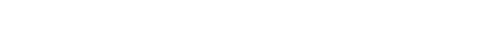glEdgeFlag.3glOpenGL man pages

```

```

## Name

```  glEdgeFlag, glEdgeFlagv - flag edges as either boundary or nonboundary

```

## C Specification

```  void glEdgeFlag( GLboolean flag )

```

## Parameters

```
flag	Specifies the current edge flag	value, either true or false.

```

## C Specification

```  void glEdgeFlagv( const GLboolean *flag )

```

## Parameters

```
flag Specifies a pointer to an array that contains a single Boolean
element,	which replaces the current edge	flag value.

```

## Description

```  Each vertex of a polygon, separate triangle, or separate quadrilateral
specified between a glBegin/glEnd pair is marked as the start	of either a
boundary or nonboundary edge.	 If the	current	edge flag is true when the
vertex is specified, the vertex is marked as the start of a boundary edge.
Otherwise, the vertex	is marked as the start of a nonboundary	edge.
glEdgeFlag sets the edge flag	to true	if flag	is nonzero, false otherwise.

The vertices of connected triangles and connected quadrilaterals are always
marked as boundary, regardless of the	value of the edge flag.

Boundary and nonboundary edge	flags on vertices are significant only if
GL_POLYGON_MODE is set to GL_POINT or	GL_LINE.  See glPolygonMode.

Initially, the edge flag bit is true.

```

## Notes

```  The current edge flag	can be updated at any time.  In	particular,
glEdgeFlag can be called between a call to glBegin and the corresponding
call to glEnd.

```

## Associated Gets

```  glGet	with argument GL_EDGE_FLAG

```

```  glBegin, glPolygonMode